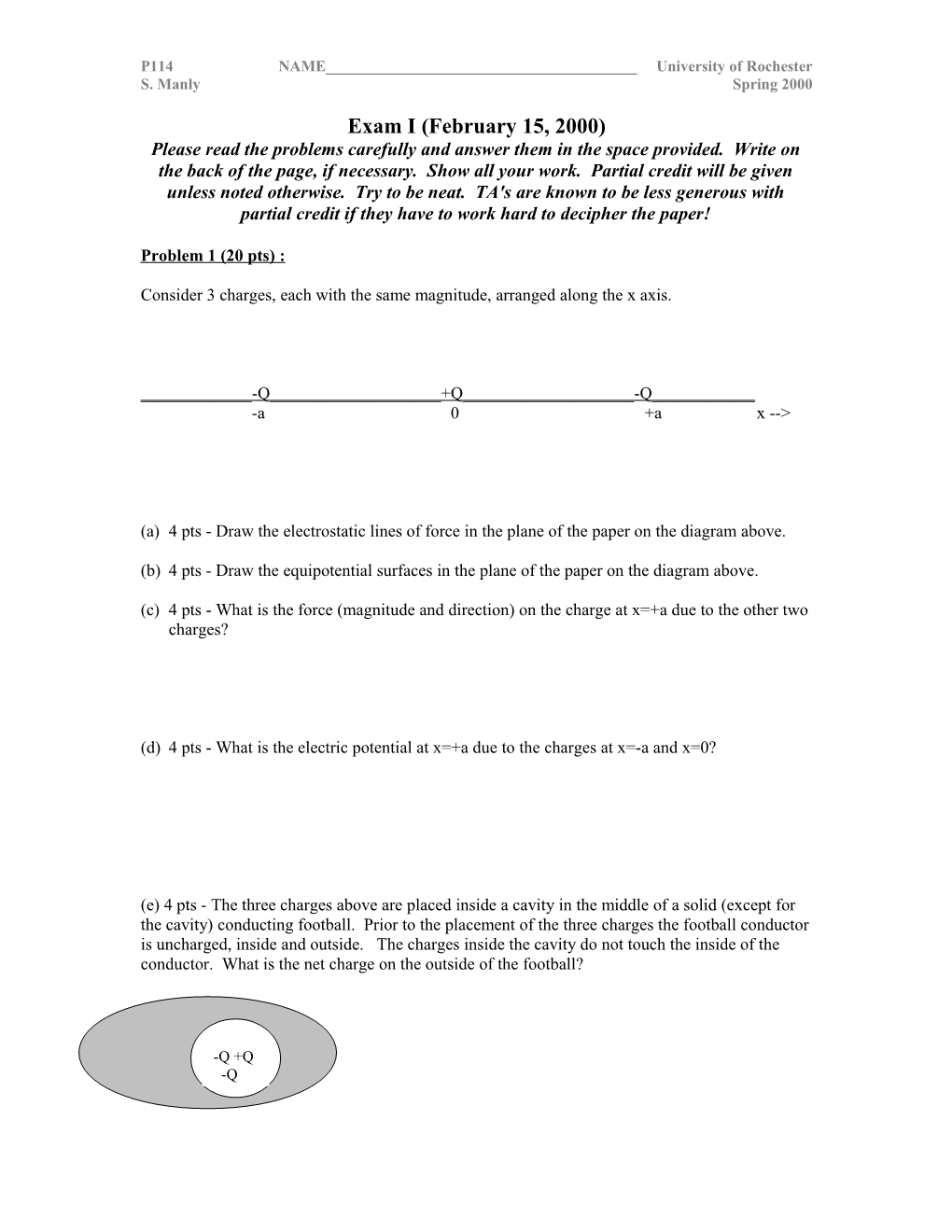# Consider 3 Charges, Each with the Same Magnitude, Arranged Along the X AxisP114 NAME______University of Rochester

S. ManlySpring 2000

Exam I (February 15, 2000)

Please read the problems carefully and answer them in the space provided. Write on the back of the page, if necessary. Show all your work. Partial credit will be given unless noted otherwise. Try to be neat. TA's are known to be less generous with partial credit if they have to work hard to decipher the paper!

Problem 1 (20 pts) :

Consider 3 charges, each with the same magnitude, arranged along the x axis.

______-Q______+Q______-Q______

-a 0+ax -->

(a)4 pts - Draw the electrostatic lines of force in the plane of the paper on the diagram above.

(b)4 pts - Draw the equipotential surfaces in the plane of the paper on the diagram above.

(c)4 pts - What is the force (magnitude and direction) on the charge at x=+a due to the other two charges?

(d)4 pts - What is the electric potential at x=+a due to the charges at x=-a and x=0?

(e) 4 pts - The three charges above are placed inside a cavity in the middle of a solid (except for the cavity) conducting football. Prior to the placement of the three charges the football conductor is uncharged, inside and outside. The charges inside the cavity do not touch the inside of the conductor. What is the net charge on the outside of the football?

Problem 2 (20 pts, zero/half/full credit) :

Arguably, the most influential set of experiments in the development of the atomic model took place in Rutherford's laboratory. In these experiments, a stream of alpha particles (a charged form of radiation) were scattered off gold atoms in a thin foil. The distribution of the scattered particles provided evidence that the positive charge in the gold atom was concentrated (effectively) at a point, while the negative charge in the gold atom was distributed in a large sphere about the positive "nucleus". For Rutherford to come to such a conclusion, he had to calculate the electric field produced by different configurations of the positive and negative charge in the gold atom and the effect of such electric fields on the approaching alpha particle.

Your job is to determine the electric field inside a gold atom. Consider the following model of the gold atom: (a) the positive charge of +Z|e| is concentrated at a point (Z is the atomic number of gold, |e| = the magnitude of the electron or proton charge), (b) the negative charge of -Z|e| uniformly fills a sphere with radius a centered on the point of positive charge. Circle the expression that describes the magnitude of the electric field in the region 0<r<a. You must show work that supports your conclusion to receive credit.

(a) (b)(c)(d)

(e)(f)(g)

Problem 3 (20pts,) :

(a)10 pts - A thin stream of water emanates from the tip of a pipette. If a negatively charged rod is brought near the stream of water, the stream is attracted toward the charged rod. Why? What happens if a positively charged rod is brought near the same stream?

(b) 10 pts - An electric dipole is placed in a nonuniform electric field (such as that surrounding a point charge). Is there a net force on the dipole? Why?

Problem 4 (20 pts) :

A large conducting sphere of radius R is connected to a small conducting sphere of radius r via a conducting wire as shown in the figure below. A total charge Qtotal is placed on the two-sphere-wire configuration. That charge distributes itself around the configuration such that the large sphere has a charge of Q on it and the small sphere has a charge of q on it. (Qtotal = Q + q)

(a)5 pts - What is the magnitude of the electric field inside the large sphere?

(b)5 pts - How much energy would be required to move a small positive test charge from the small sphere to the large sphere?

(c)10 pts - What is the ratio of charge on the small sphere (q) to the charge on the large sphere (Q) in terms of the radii of the spheres?

Problem 5(20pts) :

I have a dear friend named Chaz who was hired as a consultant by the State Police to figure a way to deal with cars exceeding the speed limit near East river road on I-390. After they rejected his proposals of random oil slicks and heat-seeking missiles, Chaz came up with an idea that the police are considering. They want your help evaluating the idea.

Chaz proposes equipping two overpasses separated by 100 meters with large conducting plates such that they look effectively like an infinite parallel plates to cars passing through on the road. Cars pass through a hole in one plate (the first overpass they encounter) and proceed between the plates until they pass through a hole in the second plate (the second overpass). Chaz intends to place a charge per unit area of =+1.1x10-4 Coulombs/m2 on the first plate the car passes through and a charge per unit area of =-1.1x10-4 Coulombs/m2on the second plate. As a car comes speeding through the first plate, a wire brushdeposits a charge of -0.001 Coulombs on the car.

(a)7 pts - Whatis the difference in potential between the two plates (overpasses)?

(b)7 pts - How much energy does the car lose as it passes between the two overpasses due to Chaz's device?

(c)6 pts- Assuming the car has a mass of 1500 kg and an initial speed of 100 mph = 170 km/hour = 47.2 m/s, what is the final speed of the car? Ignore friction and any action the driver might take, such as braking or giving the car gas … i.e., consider only the change in speed due to Chaz's device.

Chaz and law enforcement officials everywhere thank you for your help